# Go Math Grade 4 Answer Key Homework FL Chapter 9 Relate Fractions and Decimals Review/Test

Self study is possible with Go Math Grade 4 Answer Key Homework FL Chapter 9 Relate Fractions and Decimals Review/Test. Start your practice now by referring to Go Math 4th Grade Answer Key Homework FL Chapter 9 Relate Fractions and Decimals Review/Test. Every problem is explained in a detailed way to help the 4th standard students to practice in the best way.

## Go Math Grade 4 Answer Key Homework FL Chapter 9 Relate Fractions and Decimals Review/Test

### Review/Test – Page No. 373

Choose the best term from the box.Question 1.
One of ten equal parts is one __________.
_______

One of ten equal parts is one-tenth.

Question 2.
A __________ is a symbol used to separate dollars from cents in money amounts and to separate the ones and the tenths places in decimals.
_______

A decimal point is a symbol used to separate dollars from cents in money amounts and to separate the ones and the tenths places in decimals.

Question 3.
A ________________ is a number with one or more digits to the right of the decimal point.
_______

A decimal  is a number with one or more digits to the right of the decimal point.

Write the fraction and the decimal shown by the model.

Question 4.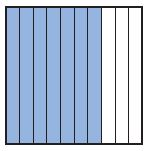$$\frac{□}{□}$$

Answer: $$\frac{7}{10}$$

Explanation:
We can see from the above figure that there are 10 blocks and among them, 7 are shaded. So, the fraction of the shaded part is $$\frac{7}{10}$$

Question 4.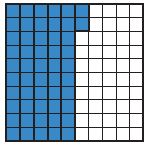$$\frac{□}{□}$$

Answer: $$\frac{52}{100}$$

Explanation:
We can see from the above figure that there are 100 boxes, in which 52 are shaded. So, the fraction of the shaded part is $$\frac{52}{100}$$

Write the number as hundredths in fraction form and decimal form.

Question 6.
$$\frac{9}{10}$$
Type below:
________

Explanation:
The hundredth of the fraction $$\frac{9}{10}$$ is $$\frac{90}{100}$$. And the decimal form of the given fraction is 0.9

Question 7.
$$\frac{3}{10}$$
Type below:
________

Explanation:
The hundredth of the fraction $$\frac{3}{10}$$ is $$\frac{30}{100}$$. And the decimal form of the given fraction is 0.3.

Question 8.
0.2
Type below:
________

Answer: $$\frac{2}{10}$$

Explanation:
The hundredth of the fraction $$\frac{2}{10}$$ is $$\frac{20}{100}$$. And the decimal form of the given fraction is 0.2

Find the sum.

Question 9.
$$\frac{5}{10}+\frac{30}{100}$$ = $$\frac{□}{□}$$

Answer: $$\frac{80}{100}$$

Explanation:
Given the fractions 5/10 and 30/100
The denominators of both the fractions are different. Make the denominators common.
$$\frac{5}{10}$$ × $$\frac{10}{10}$$ = $$\frac{50}{100}$$
$$\frac{50}{100}$$ + $$\frac{30}{100}$$ = $$\frac{80}{100}$$

Question 10.
$$\frac{6}{10}+\frac{4}{100}$$ = $$\frac{□}{□}$$

Answer: $$\frac{64}{100}$$

Explanation:
Given the fractions 6/10 and 4/100
The denominators of both the fractions are different. Make the denominators common.
$$\frac{6}{10}$$ × $$\frac{10}{10}$$ = $$\frac{60}{100}$$
$$\frac{60}{100}$$ + $$\frac{4}{100}$$ = $$\frac{64}{100}$$
$$\frac{6}{10}+\frac{4}{100}$$ = $$\frac{64}{100}$$

Question 11.
0.24 + 0.1 = _____

Explanation:
0.1 = 0.10
0.24 + 0.10 = 0.34

Compare. Write <, >, or =.

Question 12.
3.45 _____ 3.54

Explanation:
The decimal 3.45 is less than 3.54

Question 13.
1.7 _____ 1.70

Explanation:
The decimal 1.7 and 1.70 are same. Thus 1.7 = 1.70

Question 14.
8.1 _____ 8.01

Explanation:
8.1 is greater than 8.01

Question 15.
$4.25 _____$3.75

Explanation:
$4.25 is greater than$3.75

### Review/Test – Page No. 374

Question 16.
Which fraction or mixed number and decimal is shown by the model?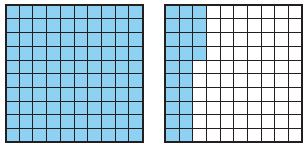Options:
a. $$\frac{24}{100}$$, 0.24
b. 1 $$\frac{24}{100}$$, 1.24
c. 1 $$\frac{76}{100}$$, 1.76
d. 1 $$\frac{24}{10}$$, 1.24

Answer: 1 $$\frac{24}{100}$$, 1.24

Explanation:
There are 100 blocks in each box. In that 124 blocks are shaded. So, the mixed fraction of the shaded part is 1 $$\frac{24}{100}$$, 1.24
Thus the correct answer is option b.

Question 17.
Bethany collected 0.7 inch of rain in her rain gauge. How many hundredths of an inch did she collect?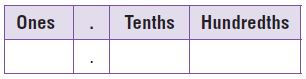Options:
a. $$\frac{7}{100}$$
b. $$\frac{7}{10}$$
c. $$\frac{70}{100}$$
d. $$\frac{7}{1}$$

Answer: $$\frac{7}{10}$$

Explanation:
The fraction of the decimal 0.7 is $$\frac{7}{10}$$
Thus the correct answer is option b.

Question 18.
Pam paid for her lunch with the amount of money shown below.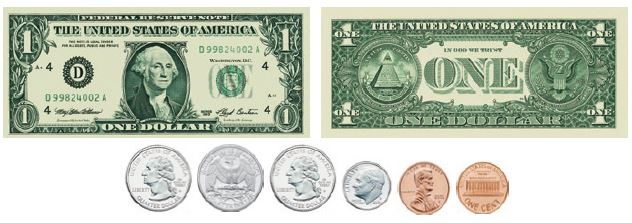How much money did she spend?
Options:
a. 2 $$\frac{62}{100}$$ dollars
b. 2 $$\frac{77}{100}$$ dollars
c. 2 $$\frac{87}{100}$$ dollars
d. 3 $$\frac{2}{100}$$ dollars

Answer: 2 $$\frac{62}{100}$$ dollars

### Review/Test – Page No. 375

Question 19.
Carson shaded a model to represent the part of his book he read this weekend. Which decimal represents the part of the book he read?Options:
a. 4.0
b. 0.44
c. 0.4
d. 0.04

Explanation:
There are 10 blocks and among them, 4 are shaded. Thus the decimal form of the shaded part is 0.4.
Thus the correct answer is option c.

Question 20.
Christelle is making a doll house. The doll house is $$\frac{6}{10}$$ meter high without the roof. The roof is $$\frac{15}{100}$$ meter high. What will the height of the doll house be, with the roof?
Options:
a. $$\frac{21}{100}$$ meter
b. $$\frac{75}{100}$$ meter
c. 1 $$\frac{6}{100}$$ meter
d. $$\frac{60}{100}$$ meter

Answer: $$\frac{75}{100}$$ meter

Explanation:
Given,
Christelle is making a doll house. The doll house is $$\frac{6}{10}$$ meter high without the roof.
The roof is $$\frac{15}{100}$$ meter high.
$$\frac{6}{10}$$ and $$\frac{15}{100}$$ the denominators are different. So make the denominators equal first.
$$\frac{6}{10}$$ × $$\frac{10}{10}$$ = $$\frac{60}{100}$$
$$\frac{60}{100}$$ + $$\frac{15}{100}$$ = $$\frac{75}{100}$$ meter
Thus the correct answer is option b.

Question 21.
Amie has three quarters and one nickel. If she and three girls share the money equally, what will each person get?
Options:
a. $0.10 b.$0.15
c. $0.20 d.$0.25

Answer: $0.25 Explanation: 1 quarter =$0.25
3 quarters = 3 × $0.25 =$0.75
1 nickel = $0.05$0.75 + $0.05 =$0.80
If she and three girls share the money equally = $0.80/3 =$0.25
Thus the correct answer is option d.

### Review/Test – Page No. 376

Question 22.
There is $$\frac{30}{100}$$ of a liter of orange juice in one container and $$\frac{5}{10}$$ of a liter of pineapple juice in another container. If Mrs. Morales combines the two juices, how much orange-pineapple juice will she have? Explain how you found your answer.
Type below:
________

The total quantity was found by adding the quantities of individual kinds of juice. The addition was performed by expressing each fraction using the common denominator of 10, then reducing the final result.
$$\frac{30}{100}$$ + $$\frac{5}{10}$$
= $$\frac{30}{100}$$ + $$\frac{50}{100}$$
= $$\frac{80}{100}$$
= $$\frac{4}{5}$$

Question 23.
Write the amount of orange-pineapple juice as a decimal.
_____

$$\frac{4}{5}$$
= 0.8
Thus the amount of orange-pineapple juice as a decimal is 0.8

Question 24.
Luke lives 0.4 kilometer from a skating rink. Mark lives 0.25 kilometer from the skating rink.
A. Who lives closer to the skating rink? Explain.
Type below:
________

Let’s take a look at their decimal places.
For 0.4, the four is in the tenths place, therefore it’s 4/10
For 0.25, the number ends in the hundredths place, therefore it’s 25/100
To compare them, I can make the 4/10 out of 100 and we’ll see which has the larger denominator.
To do this, we multiply 4/10 by 10/10 to get 40/100.
40/100 is greater than 25/100, so Luke lives closer to the skating rink.

Question 24.
B. How can you write each distance as a fraction? Explain.
Type below:
________

For 0.4, the four is in the tenths place, therefore it’s 4/10
For 0.25, the number ends in the hundredths place, therefore it’s 25/100

Question 24.
C. Luke is walking to the skating rink to pick up a practice schedule. Then he is walking to Mark’s house. Will he walk more than a kilometer or less than a kilometer? Explain.
Type below:
________